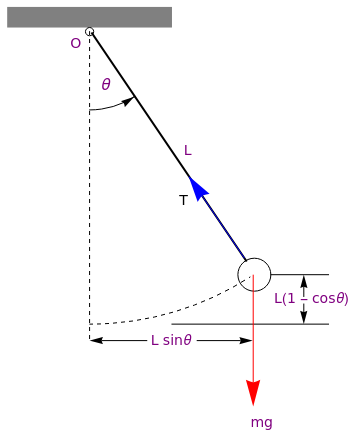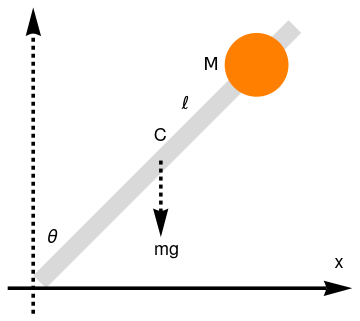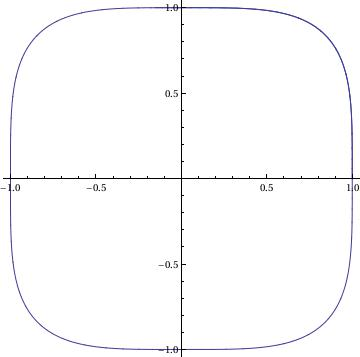Introduction to Linear Algebra with Mathematica

# Preface

This is devoted to the fasinated topic---pendulum equations.

# Pendulum Equations

A simple pendulum consists of a single point of mass m (bob) attached to a rod (or wire) of length $$\ell$$ and of negligible weight. We denote by θ the angle measured between the rod and the vertical axis, which is assumed to be positive in counterclockwise direction. We consider the simple pendulum that is characterized by the following assumptions:
• The bob is free to move within a plane (so we consider only two-dimensional oscillations).
• The system is conservative, so the pendulum rotates in a vacuum and friction in the pivot is negligible.
• The bob of mass m is attached to one end of a rigid, but weightless rod of length ℓ, which is assumed to be constant during the pendulum motion. The other end of the rod (or rigid spring) is supported at the pivot.
First, we illustrate with Mathematica the motion of the simple pendulum. Introducing the function f for circle arrow
start = -$Pi]/2; \[Theta] = start + Pi/6; f[s_Circle] := s /. Circle[a_, r_, {start_, end_}] :> ({s, Arrow[{# - r/10^6 {-Sin@end, Cos@end}, #}]} &[ a + r {Cos@end, Sin@end}]) we proceed arc = Graphics@f[Circle[{0, 0}, 1, {-Pi/2, -1.0}]]; polygon = Polygon[{{-1, 0}, {1, 0}, {1, 1/4}, {-1, 1/4}}]; top = Graphics[{Gray, polygon}]; circle1 = Graphics[Circle[{0, -1/20}, 1/20]]; circle2 = Graphics[Circle[{2, -3}, 1/5]]; circle3 = Graphics[{Dashed, Circle[{0, 0}, 3.6, {-1.57, -1.0}]}]; line1 = Graphics[{Dashed, Line[{{0, -1/20}, {0, -3.8}}]}]; line2 = Graphics[{Thick, Line[{{0.03, -0.07}, {1.9, -2.83}}]}]; arrow = Graphics[{Red, Arrowheads[0.08], Arrow[{{1.986, -3.0}, {1.986, -4.6}}]}]; line3 = Graphics[{Line[{{2.2, -3.8}, {2.9, -3.8}}]}]; arrow2a = Graphics[{Arrowheads[0.04], Arrow[{{0.7, -3.8}, {0, -3.8}}]}]; arrow2b = Graphics[{Arrowheads[0.04], Arrow[{{1.3, -3.8}, {1.98, -3.8}}]}]; text1 = Graphics[ Text[Style["L sin\[Theta]", FontSize -> 14, Purple], {1.0, -3.8}]]; text2 = Graphics[ Text[Style["mg", FontSize -> 14, Purple], {2.1, -4.8}]]; line3 = Graphics[{Line[{{1.0, -3.6}, {2.9, -3.6}}]}]; line4 = Graphics[{Line[{{2.2, -3.0}, {2.9, -3.0}}]}]; text3 = Graphics[ Text[Style["L(1 - cos\[Theta])", FontSize -> 14, Purple], {2.7, -3.3}]]; arrow3a = Graphics[{Arrowheads[0.04], Arrow[{{2.6, -3.35}, {2.6, -3.6}}]}]; arrow3b = Graphics[{Arrowheads[0.04], Arrow[{{2.6, -3.27}, {2.6, -3.0}}]}]; text4 = Graphics[ Text[Style["L", FontSize -> 14, Purple], {1.2, -1.5}]]; text5 = Graphics[ Text[Style["\[Theta]", FontSize -> 16, Purple], {0.2, -0.7}]]; text6 = Graphics[ Text[Style["O", FontSize -> 14, Purple], {-0.16, -0.2}]]; textT = Graphics[ Text[Style["T", FontSize -> 14, Black], {1.15, -2.1}]]; arrowT = Graphics[{Blue, Arrowheads[0.08], Arrow[{{1.88, -2.8}, {1.2, -1.8}}]}]; Show[top, circle1, circle2, circle3, line1, line2, line3, arrow, arrow2a, arrow2b, text1, text2, line4, text3, arrow3a, arrow3b, text4, text5, text6, arrowT, textT, arc]The modeling of the motion is greatly simplified when the given body (bob) is considered essentially as a point particle. The position of the bob is described by the angle θ between the rod and the downward equilibrium vertical position, with the counterclockwise direction taken as positive. The only force acting on the pendulum is the gravitational force m g, acting downward, where g denotes the acceleration due to gravity. The position of the bob can be determined in Cartesian coordinates as \[ x = \ell \,\sin \theta , \qquad y = -\ell\,\cos\theta ,$ where the origin is taken at the pivot and the positive vertical direction is upward. Using $${\cal L} = \mbox{K} - \Pi ,$$ the Lagrangian, which is the difference of the kinetic energy K and the potential energy Π of the system, we have $\frac{\text d}{{\text d}t} \,\frac{\partial {\cal L}}{\partial \dot{\theta}} = \frac{\partial {\cal L}}{\partial \theta} .$

With the kinetic energy expressed via the angular displacement θ
$\mbox{K} = \frac{m}{2} \left( \dot{x}^2 + \dot{y}^2 \right) = \frac{m}{2}\, \ell^2 \left[ \left( \dot{\theta} \,\cos\theta \right)^2 + \left( \dot{\theta} \,\sin \theta \right)^2 \right] = \frac{m}{2}\,\ell^2 \dot{\theta}^2 .$
and the potential energy
$\Pi = mg \left( \ell + y \right) = mg\ell \left( 1- \cos \theta \right) ,$
we derive the corresponding derivatives
$\frac{\partial \mbox{K}}{\partial \dot{\theta}} = I_0 \dot{\theta} = m\ell^2 \dot{\theta} , \qquad \frac{\partial \Pi}{\partial \theta} = mgy = mg\ell \, \sin \theta .$
Using these expressions, we obtain from the Euler--Lagrange equation $$\displaystyle \frac{\text d}{{\text d} t} \left( \frac{\partial {\cal L}}{\partial \dot{\theta}} \right) = \frac{\partial {\cal L}}{\partial \theta} ,$$ for the Lagrangian $${\cal L} = \mbox{K} - \Pi ,$$ the pendulum equation in a vacuum:
$\ddot{\theta} + \left( g/\ell \right) \sin \theta =0 \qquad \mbox{or} \qquad \ddot{\theta} + \omega_0^2 \sin \theta =0 \qquad \left( \omega_0^2 = g/\ell \right) ,$
where $$\ddot{\theta} = {\text d}^2 \theta / {\text d}t^2$$ , $$\omega_0 = \sqrt{g/\ell} >0 ,$$ and g is gravitational acceleration.

Further simplification is available by normalization, which leads to $$\omega = 1$$ and we get

$\ddot{\theta} + \sin \theta =0 .$
In practice, it is easier to study an ordinary differential equation as a system of equations involving only the first derivatives.
$\dot{x} = y, \qquad \dot{y} = -\sin x .$
The variables x and y can be interpreted geometrically. Indeed, the angle x = θ corresponds to a point on a circle whereas the velocity $$y = \dot{\theta}$$ corresponds to a point on a real line. Therefore, the set of all states (x ,y) can be represented by a cylinder, the product of a circle by a line. More formally, the phase space of the pendulum is the cylinder $$S^1 \times \mathbb{R} ,$$ its elements are couples (position,velocity).

Thus, at each point (x ,y) in the phase space, there is an attached vector $$(\dot{x}, \dot{y} ) = (y, - \sin x) .$$ This can be geometrically represented as a vector field on the cylindrical phase space.

Example: The swinging mass m has a kinetic energy of $$m \ell^2 \left( {\text d}\theta /{\text d}t \right)^2 /2$$ and a potential energy of $$mg\ell\left( 1 - \cos \theta \right) ;$$ the potential energy is zero for θ = 0. Let θM denote the maximum amplitude of the pendulum. Since dθ/dt = 0 at θ = θM, conservation of energy gives

$\frac{1}{2} \,m\ell^2 \left( \frac{{\text d}\theta}{{\text d}t} \right)^2 = mg\ell\,\cos \theta - mg\ell\,\cos \theta_M .$
Solving for the velocity dθ/dt, we obtain
$\frac{{\text d}\theta}{{\text d}t} = \pm \left( \frac{2g}{\ell} \right)^{1/2} \left( \cos \theta - mg\ell\,\cos \theta_M \right)^{1/2} ,$
with the mass m canceling out. We take t to be zero, when θ = 0 and dθ/dt > 0. An integration from θ = 0 t0 θM yields
\begin{equation} \int_0^{\theta_M} \left( \cos \theta - mg\ell\,\cos \theta_M \right)^{-1/2} {\text d}\theta = \left( \frac{2g}{\ell} \right)^{1/2} \int_0^t {\text d}t = \left( \frac{2g}{\ell} \right)^{1/2} t . \label{pendulum.1} \end{equation}
This is one-fourth of a cycle, and therefore the time t is one-fourth of the period, T. We note that θ < θM, and with a bit of clairvoyance we try the half-angle substitution
$\sin \frac{\theta}{2} = \sin \left( \frac{\theta_M}{2} \right) \sin \phi .$
With this, Eq.\eqref{pendulum.1} becomes
\begin{equation} T = 2\pi \left( \frac{\ell}{g} \right)^{1/2} \int_0^{\pi} \left[ 1 - \sin^2 \left( \frac{\theta_M}{2} \right) \sin^2 \phi \right]^{-1/2} {\text d}\phi . \label{pendulum.2} \end{equation}
Although not an obvious improvement over Eq.\eqref{pendulum.1}, the integral \eqref{pendulum.2} now defines the complete elliptic integral of the first kind,$$K \left( \sin^2 \theta_M /2 \right) .$$ From the series expansion, the period of our pendulum may be developed as a power series—powers of sin θM/2:
$T = 2\pi \left( \frac{\ell}{g} \right)^{1/2} \left\{1 + \frac{1}{4}\,\sin^2 \frac{\theta_M}{2} + \frac{9}{64}\,\sin^4 \frac{\theta_M}{2} + \cdots \right\} .$
■

# Pendulum Equation with Resistance

We convert the pendulum equation with resistance
$m\ell^2 \ddot{\theta} + c\,\dot{\theta} + mg\ell \,\sin \theta =0 .$
to a system of two first order equations by letting $$x= \theta \quad\mbox{and} \quad y = \dot{\theta} :$$
$\frac{{\text d} x}{{\text d}t} = y , \qquad \frac{{\text d} y}{{\text d}t} = -\omega^2 \sin x - \gamma \,y .$
Here $$\gamma = c/(m\,\ell ) , \ \omega^2 = g/\ell$$ are positive constants. Therefore, the above system of differential equations is autonomous. Setting $$\gamma = 0.25 \quad\mbox{and}\quad \omega^2 =1 ,$$ we ask Mathematica to provide a phase portrait for the pendulum equation with resistance:

# Pendulum with a ball

Let us consider a rod of length ℓ of mass m with attached ball of mass M at a distance L from the pivot.rod = Graphics[{LightGray, Polygon[{{0.1, 0}, {0, 0.1}, {2.0, 2.1}, {2.1, 2.0}}]}]; ball = Graphics[{Orange, Disk[{1.75, 1.75}, 0.25]}]; ar3 = Graphics[{Black, Dashed, Thickness[0.01], Arrowheads[0.08], Arrow[{{1, 1}, {1, 0.4}}]}]; ar = Graphics[{Black, Thickness[0.01], Arrowheads[0.08], Arrow[{{-0.2, 0}, {2.5, 0}}]}]; ar2 = Graphics[{Black, Dashed, Thickness[0.01], Arrowheads[0.08], Arrow[{{0, -0.2}, {0, 2.2}}]}]; tl = Graphics[{Black, Text[Style["$ScriptL]", 18, FontFamily -> "Mathematica1"], {1.2, 1.45}]}]; tx = Graphics[{Black, Text[Style["x", 18], {2.4, 0.2}]}]; tm = Graphics[{Black, Text[Style["M", 18, FontFamily -> "Mathematica1"], {1.4, 1.75}]}]; t1 = Graphics[{Black, Text[Style["C", 18], {1.0, 1.2}]}]; t2 = Graphics[{Black, Text[Style["\[Theta]", 18], {0.15, 0.4}]}]; t3 = Graphics[{Black, Text[Style["mg", 18], {1.05, 0.3}]}]; Show[rod, ar, ar2, ar3, tx, tl, tm, t1, t2, t3, ball] Rigid pendulum with a ball. Mathematica code To analyze the problem of falling meterstick of length ℓ with attached heavy weight at a distance L from the pivot, we use the tourque equation: \[ I\,\ddot{\theta} = \tau$
is the torque. This yields
$I\,\ddot{\theta} = \left( \frac{m}{2}\,g\,\ell + Mg\,L \right) \sin\theta ,$
where θ = θ(t) is the instanteneous angle of the rod with the vertical axis, and $$\displaystyle I = \frac{m}{3}\,\ell^2 + M\,L^2$$ is the moment of inertia about the lower end. Introducing the dimensionless ratios k = M/m and q = L/ℓ, we can express the angular acceleration of the loaded stick in terms of the angular acceleration of the bare meterstick and a dimensionless factor:
$\ddot{\theta} = \frac{3g}{2\ell}\cdot \frac{1 + 2kq}{1 + kq^2} \cdot \sin\theta .$
It is instructive to examine the dimensionless factor in the previous equation of motion. For q = ⅔, it equals 1 for all values of k. Adding any point mass at ⅔ of the length ℓ of a uniform rod leaves the angular acceleration and, therefore, the time of fall unchanged. For q < ⅔, the factor becomes greater than 1, and the time of fall gets shorter. The opposite is true for q > ⅔. k*

# Spherical Pendulum

Consider the motion under gravity of a bob of mass m attached to a fixed point by an inextensible massless rod of length ℓ. The bob is free to move on a sphere of radius ℓ. Using spherical coordinates (angles) θ and ϕ as the generalized coordinates, the kinetic and potential energies become
\begin{align*} \mbox{K} &= \frac{m}{2}\,\ell^2 \left( \dot{\theta}^2 + \dot{\phi}^2 \sin^2 \theta \right) , \\ \Pi &= mg\ell \left( 1- \cos \theta \right) . \end{align*}
This allows us to derive the Euler--Lagrange equations for the corresponding Lagrangian:
$\frac{\text d}{{\text d}t} \left( \frac{\partial {\cal L}}{\partial \dot{\theta}} \right) - \frac{\partial {\cal L}}{\partial \theta} = 0 , \qquad \frac{\text d}{{\text d}t} \left( \frac{\partial {\cal L}}{\partial \dot{\phi}} \right) - \frac{\partial {\cal L}}{\partial \phi} = 0 .$
Substituting the expressions for the kinetic and potential energies, we obtain two coupled equations
$\begin{split} \ddot{\theta} + \frac{g}{\ell} \,\sin\theta - \frac{1}{2} \,\dot{\phi}^2 \sin 2\theta &= 0, \\ \ddot{\phi}\,\sin\theta + 2\dot{\phi} \dot{\theta}\,\cos\theta &= 0. \end{split}$
The last equation is a first order differential equation with respect to the derivative of ϕ, so it can be integrated
$\dot{\phi} \sin^2 \theta = c, \quad \mbox{a constant}.$
Using this equation, we obtain the following differential equation for nagle θ:
$\dot{\theta} + \frac{g}{\ell} \,\sin\theta - \frac{c^2 \cos\theta}{\sin^3 \theta} = 0.$

# Applications

Example 2: Consider a pendulum with constant torque T. Its motion is governed by the differential equation
$\ddot{\theta} + \frac{g}{\ell}\,\sin\theta = \frac{T}{m\ell^2} .$
Its equilibria are determined by solving the algebraic equation
$\sin\theta = \frac{T}{mg\ell} .$
For real roots, $$\displaystyle \frac{T}{mg\ell} < 1,$$ illustrating fold or saddle-node bifurcation Its first integral is
$\frac{y^2}{2} - \frac{g}{\ell}\,\cos x - \frac{T}{m \ell^2}\, x = \mbox{constant}.$
■
Example 3: Consider a pendulum in a plane that is rotating about a vertical axis with angular velocity ω. Its motion is governed by the differential equation
$\ddot{\theta} + \frac{g}{\ell}\,\sin\theta - \omega^2 \sin x \,\cos x = 0.$
Equilibria: y = 0, x = 0, π, and also $$\displaystyle \cos x = \frac{g}{\omega^2 \ell} .$$ For real roots, $$\displaystyle \frac{g}{\omega^2 \ell} < 1,$$ illustrating a pitchfort bifurcation. The first integral:
$\frac{y^2}{2} - \frac{g}{\ell} \,\cos x + \frac{\omega^2}{4}\.\cos 2x = \mbox{constant}.$
■
We consider a periodic movement described by the equation
$\dot{x} = -y^3 , \qquad \dot{y} = x^3 .$
F[x_, y_] := -y^3;
G[x_, y_] := x^3;
sol = NDSolve[{x'[t] == F[x[t], y[t]], y'[t] == G[x[t], y[t]],
x == 1, y == 0}, {x, y}, {t, 0, 3*Pi},
WorkingPrecision -> 20]
ParametricPlot[Evaluate[{x[t], y[t]}] /. sol, {t, 0, 3*Pi}]

Out=
{{x -> InterpolatingFunction[{{0, 9.4247779607693797154}}, <>],
y -> InterpolatingFunction[{{0, 9.4247779607693797154}}, <>]}}X[t_] := Evaluate[x[t] /. sol]
Y[t_] := Evaluate[y[t] /. sol]
fns[t_] := {X[t], Y[t]};
len := Length[fns[t]];
Plot[Evaluate[fns[t]], {t, 0, 3*Pi}]

1. Vitt, A. (Aleksandr Adolʹfovich) and Gorelik, G. (Gabriel Simonovich), Oscillations of an Elastic Pendulum as an Example of the Oscillations of Two Parametrically Coupled Linear Systems, Journal of Technical Physics, 1933, vol. 3, pp. 294–307.
Its translation from the Russian in English by Lisa Shields with an introduction by Peter Lynch is available on the web:
http://copac.jisc.ac.uk/id/15694518?style=html
http://catalogue.nli.ie/Record/vtls000058645
2. P. Pokorny, Stability condition for vertical oscillation of 3-dim heavy spring elastic pendulum, Regular and Chaotic Dynamics, June 2008, Volume 13, Issue 3, pp 155--165.
http://old.vscht.cz/mat/Pavel.Pokorny/rcd/RCD155-color.pdf
3. Pendulum Wikipedia
4. Pendulum Physics
5. Lumen Physics: Pendulum
6. Predicting the Future – An Intro to Models Described by Time Dependent Differential Equations by Christian J Howard.
7. Salas, A.H. and Castillo, J.E., Exact Solution to Duffing Equation and the Pendulum Equation, Applied Mathematical Sciences, Vol. 8, 2014, no. 176, 8781 - 8789; http://dx.doi.org/10.12988/ams.2014.44243
8. M. G. Olsson, Why does a mass on a spring sometimes misbehave?, American Journal of Physics, 44, Issue 12, 1211 (1976); https://doi.org/10.1119/1.10265
9. Casey, J., Geometrical derivation of Lagrange’s equations for a system of particles, American Journal of Physics, 62(9) 836–847 (1994). https://doi.org/10.1119/1.17470
10. Corey Zammit, Nirantha Balagopal, Zijun Li, Shenghao Xia, and Qisong Xiao, The dynamic of the elastic pendulum, Arizona.
11. Qisong Xiao; Shenghao Xia; Corey Zammit; Nirantha Balagopal; Zijun Li, Dynamics of the Elastic Pendulum, Arizona.
12. C. Madigan, The Spring Pendulum, MapleSoft.
13. K. Craig, Pring-pendulum dynamic system, NYU, 2001.